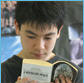International Baccalaureate

The Middle Years Programme - MYP

 Mathematics Back to MYP Course Index

Aims

The aims of teaching and learning mathematics are to encourage and enable students to:
• recognize that mathematics permeates the world around us
• appreciate the usefulness, power and beauty of mathematics
• enjoy mathematics and develop patience and persistence when solving problems
• understand and be able to use the language, symbols and notation of mathematics
• develop mathematical curiosity and use inductive and deductive reasoning when solving problems
• become confident in using mathematics to analyse and solve problems both in school and in real-life situations
• develop the knowledge, skills and attitudes necessary to pursue further studies in mathematics
• develop abstract, logical and critical thinking and the ability to reflect critically upon their work and the work of others
• develop a critical appreciation of the use of information and communication technology in mathematics
• appreciate the international dimension of mathematics and its multicultural and historical perspectives.

Objectives

#### A. Knowledge and understanding

Knowledge and understanding are fundamental to studying mathematics and form the base from which to explore concepts and develop problem-solving skills. Through knowledge and understanding students develop mathematical reasoning to make deductions and solve problems.

At the end of the course, students should be able to:

• know and demonstrate understanding of the concepts from the five branches of mathematics (number, algebra, geometry and trigonometry, statistics and probability, and discrete mathematics)
• use appropriate mathematical concepts and skills to solve problems in both familiar and unfamiliar situations including those in real-life contexts
• select and apply general rules correctly to solve problems including those in real-life contexts.

#### B. Investigating patterns

Investigating patterns allows students to experience the excitement and satisfaction of mathematical discovery. Mathematical inquiry encourages students to become risk-takers, inquirers and critical thinkers. The ability to inquire is invaluable in the MYP and contributes to lifelong learning.

Through the use of mathematical investigations, students are given the opportunity to apply mathematical knowledge and problem-solving techniques to investigate a problem, generate and/or analyse information, find relationships and patterns, describe these mathematically as general rules, and justify or prove them.

At the end of the course, when investigating problems, in both theoretical and real-life contexts, student should be able to:

• select and apply appropriate inquiry and mathematical problem-solving techniques
• recognize patterns
• describe patterns as relationships or general rules
• draw conclusions consistent with findings
• justify or prove mathematical relationships and general rules.

#### C. Communication in mathematics

Mathematics provides a powerful and universal language. Students are expected to use mathematical language appropriately when communicating mathematical ideas, reasoning and findings—both orally and in writing.

At the end of the course, students should be able to communicate mathematical ideas, reasoning and findings by being able to:

• use appropriate mathematical language (notation, symbols, terminology) in both oral and written explanations
• use different forms of mathematical representation (formulae, diagrams, tables, charts, graphs and models)
• move between different forms of representation.

Students are encouraged to choose and use ICT tools as appropriate and, where available, to enhance communication of their mathematical ideas. ICT tools can include graphic display calculators, screenshots, graphing, spreadsheets, databases, and drawing and word-processing software.

#### D. Reflection in mathematics

MYP mathematics encourages students to reflect upon their findings and problem-solving processes. Students are encouraged to share their thinking with teachers and peers and to examine different problem-solving strategies. Critical reflection in mathematics helps students gain insight into their strengths and weaknesses as learners and to appreciate the value of errors as powerful motivators to enhance learning and understanding.

At the end of the course students should be able to:

• explain whether their results make sense in the context of the problem
• explain the importance of their findings
• justify the degree of accuracy of their results where appropriate
• suggest improvements to the method when necessary.